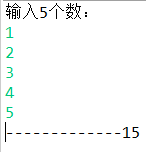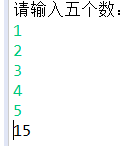# 关于返回一个整数数组中最大子数组的和的问题

2、数组中连续的一个或多个整数组成一个子数组，每个子数组都有一个和。

3、求所有子数组的和的最大值。要求时间复杂度为O(n)

package about_bank_account;

import java.util.Scanner;

public class Test {
public static void main(String[] args) {

Scanner in = new Scanner(System.in);

int[] A = new int;//大数组
int[] B = new int;
int temp = 0;
int i;
int k;//k为行
int t1 = 0;//小数组内循环
int t2 = 0;//记录小数组内数字个数
int sum = 0;//和
int j = 0;
int max = 0;

System.out.println("输入5个数：");

for(i = 0;i < 5;i++) {
temp = in.nextInt();
A[i] = temp;
}

for(k = 0;k < 15;k++) {
for(t1 = j;t1 <= t2;t1++) {
sum = sum + A[t1];
}

t2++;
B[k] = sum;
sum = 0;

if(t2 == 5) {
j = j + 1;
t2 = j;
}
}

max = B;
for(k = 0;k < 15;k++) {
if(max < B[k]) {
max = B[k];
}
}

System.out.print("-------------"+max);
}
}若原数组为：

 5 -1 -2 1 2

转化后数组为：

 5 -3 3

然后在转化后的数组的基础上，从第一个数开始，依次加两个数然后比较，加到最后一位数后，再从第二个数开始加，这样循环，找出其中最大数

（本方法我没有具体实现，转化后数组第一个数字正负不同，加的方式不同）

package about_bank_account;

import java.util.Scanner;

public class Test {

public static void main (String[] args){
@SuppressWarnings("resource")
Scanner in=new Scanner(System.in);
int num[]=new int;
int max=0;
int rmax=0;
System.out.println("请输入五个数：");
for(int i=0;i<5;i++) {
num[i]=in.nextInt();
}
rmax=num;
for(int i=0;i<5;i++) {
if(max<=0) {
max=num[i];
}else {
max+=num[i];
}
if(rmax<max) {
rmax=max;
}
}
System.out.println(rmax);
}

}04-193万+
05-038607-145275
02-25777
02-25431
10-05484
04-242935
03-1728
11-231986
11-30102
11-29323
11-29638
11-30248
11-27841
11-28238
11-3090
11-26372
11-29161
11-3094点击重新获取扫码支付余额充值